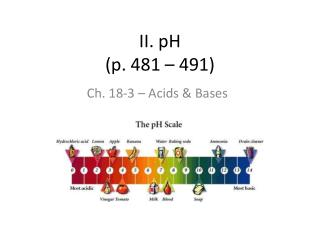DownloadDownload PresentationII. pH (p. 481 – 491)

# II. pH (p. 481 – 491)

Download Presentation## II. pH (p. 481 – 491)

- - - - - - - - - - - - - - - - - - - - - - - - - - - E N D - - - - - - - - - - - - - - - - - - - - - - - - - - -
##### Presentation Transcript

1. II. pH(p. 481 – 491) Ch. 18-3 – Acids & Bases

2. Self-Ionization of Water • Two water molecules produce a hydronium ion and a hydroxide ion by transfer of a proton: H2O + H2O H3O+ + OH- • The concentrations of H3O+ and OH- in pure water are each 1.0 x 10-7 mol/L at 25oC • Concentration is represented by [ ] Ex. [H3O+] means “hydronium ion concentration in moles per liter” So… [H3O+] = 1.0 x 10-7M and [OH-] = 1.0 x 10-7M

3. Self-Ionization of Water Cont… • Since the product of these ions remains the same in water and dilute aqueous solutions at constant temperature, we have what is called “the ionization constant of water”: Kw = [H3O+][OH-] = 1.0 x 10-14M2 • Recall that strong acids and strong bases dissociate completely in aqueous solutions. So, we can say that one mole of a strong acid will yield one mole of H3O+, and one mole of a strong base will yield one mole of OH-. • We can use this information to find the concentration of these ions in different solutions.

4. Practice • Find the hydroxide ion concentration of 3.0 x 10-2M HCl. [H3O+][OH-] = 1.0 x 10-14 [3.0 x 10-2][OH-] = 1.0 x 10-14 [OH-] = 3.3 x 10-13M • Acidic or basic? Acidic • How do you know?...

5. pH Scale 0 14 7 Increasing Neutral Increasing Acidity Basicity • Useless Fact! – pH stands for pouvior hydrogene, meaning “hydrogen power.” • pH is defined as the negative of he common logarithm of the hydronium ion concentration, or simply: pH = -log[H3O+] • If a neutral solution has a [H3O+] of 1 x 10-7, then: pH = -log(1 x 10-7) = - (-7.0) = 7.0

6. pH Scale Continued… • Likewise, pOH is defined as the negative of the natural logarithm of the hydroxide ion concentration, or simply: pOH = -log[OH-] • If a neutral solution has a [OH-] of 1 x 10-7, then: pOH = -log(1 x 10-7) = -(-7.0) = 7.0 • Since [H3O+] gives us pH, and [OH-] gives us pOH, and both are related by Kw: Kw = [H3O+][OH-] = 1 x 10-14, -log(Kw) = -log(1 x 10-14) = 14.0 • Therefore, we can say that: pH + pOH = 14.0

7. pH of Some Common Substances

8. Summary of Equations • Kw = [H3O+][OH-] = 1 x 10-14 • pH = -log[H3O+] • pOH = -log[OH-] • pH + pOH = 14.0

9. Practice • What is the pH of 0.050M HNO3? pH = -log[H3O+] pH = -log[0.050] pH = 1.3 • Acidic or Basic? Acidic

10. Practice • What is the molarity of HBr in a solution that has a pOH of 9.6? * We want the molarity of HBR, which is an acid, so we want to start with finding the pH: pH + pOH = 14 pH + 9.6 = 14 pH = 4.4 * Next we can use the pH equation to find [H3O+]: pH = -log[H3O+] 4.4 = -log[H3O+] -4.4 = log[H3O+] (use the inverse log function on your calculator to find this) [H3O+] = 4.0 x 10-5M HBr